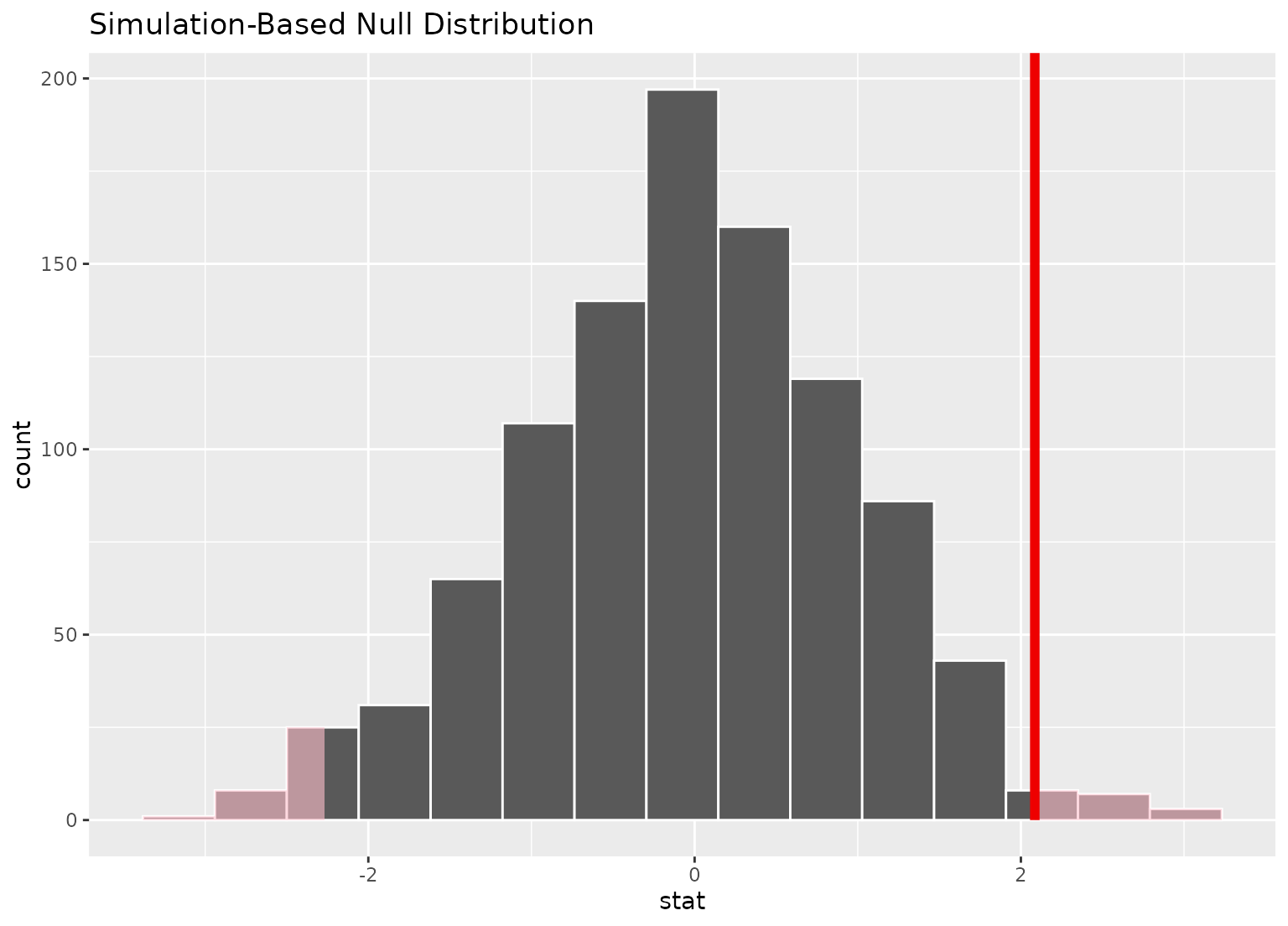shade_p_value() plots p-value region(s) (using "area under the curve" approach) on top of the visualize() output. It should be used as \ggplot2\ layer function (see examples). shade_pvalue() is its alias.

Learn more in vignette("infer").

shade_p_value(obs_stat, direction, color = "red2", fill = "pink", ...)

shade_pvalue(obs_stat, direction, color = "red2", fill = "pink", ...)

## Arguments

obs_stat A numeric value or 1x1 data frame corresponding to what the observed statistic is. A string specifying in which direction the shading should occur. Options are "less", "greater", or "two-sided". Can also give "left", "right", "both", "two_sided", or "two sided". If NULL then no shading is actually done. A character or hex string specifying the color of the observed statistic as a vertical line on the plot. A character or hex string specifying the color to shade the p-value region. If NULL then no shading is actually done. Other arguments passed along to \ggplot2\ functions.

## Value

A list of \ggplot2\ objects to be added to the visualize() output.

## See also

shade_confidence_interval() to add information about confidence interval.

## Examples

# find the point estimate---mean number of hours worked per week
point_estimate <- gss %>%
specify(response = hours) %>%
calculate(stat = "mean") %>%
dplyr::pull()

# ...and a null distribution
null_dist <- gss %>%
# ...we're interested in the number of hours worked per week
specify(response = hours) %>%
# hypothesizing that the mean is 40
hypothesize(null = "point", mu = 40) %>%
# generating data points for a null distribution
generate(reps = 1000, type = "bootstrap") %>%
# finding the null distribution
calculate(stat = "mean")

# shade the p-value of the point estimate
null_dist %>%
visualize() +
shade_p_value(obs_stat = point_estimate, direction = "two-sided")# More in-depth explanation of how to use the infer package
if (FALSE) {
vignette("infer")
}